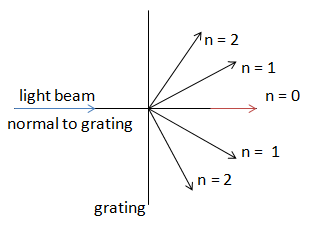# Diffraction

Diffraction refers to various phenomena which occur when a wave encounters an obstacle or a slit. It is defined as the bending of light around the corners of an obstacle or aperture into the region of geometrical shadow of the obstacle. In classical physics, the diffraction phenomenon is described as the interference of waves according to the Huygens–Fresnel principle.

the behavior of waves traveling along a rope from a more dense medium to a less dense medium (and vice versa) was discussed. The wave doesn't just stop when it reaches the end of the medium. Rather, a wave will undergo certain behaviors when it encounters the end of the medium. Diffraction also occurs when a wave passes through a gap in a barrier.a modification which light undergoes especially in passing by the edges of opaque bodies or through narrow openings and in which the rays appear to be deflected.

The variation in wave intensity can be mathematically modeled. From the center of the slit, the diffracting waves propagate radially. The angle of the minimum intensity (θmin) can be related to wavelength (λ) and the slit's width (d) such that:

dsinθmin = λ

The intensity (I) of waves at any angle can also be calculated as a relation to slit width, wavelength and intensity of the original waves before passing through the slit:

I∴ = 10(sin(πx)/(πx)2)

where x = d/λθ### General diffraction

Several qualitative observations can be made of diffraction in general:

The angular spacing of the features in the diffraction pattern is inversely proportional to the dimensions of the object causing the diffraction.The diffraction angles are invariant under scaling; that is, they depend only on the ratio of the wavelength to the size of the diffracting object.When the diffracting object has a periodic structure.

### Approximations

It is mathematically easier to consider the case of far-field or Fraunhofer diffraction, where the point of observation is far from that of the diffracting obstruction, and as a result, involves less complex mathematics than the more general case of near-field or Fresnel diffraction.

S = √(L2 + (x + (a/2)))2

If we now consider the situation where L >>(x + a / 2)

This is the Fresnel approximation. To further simplify things.